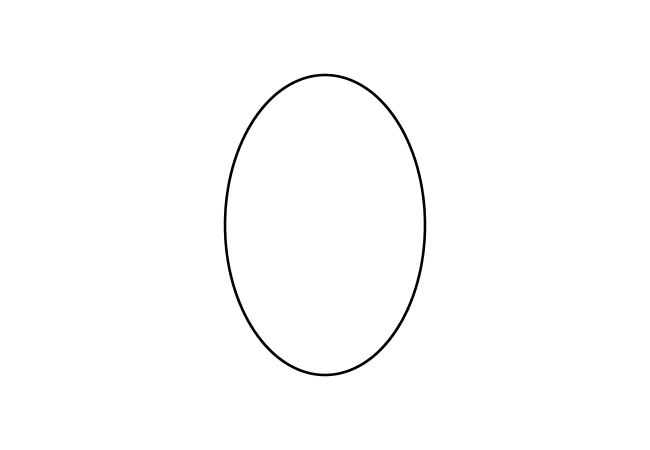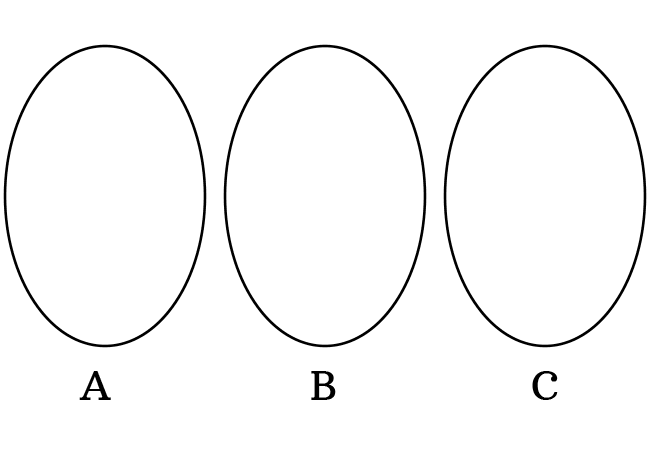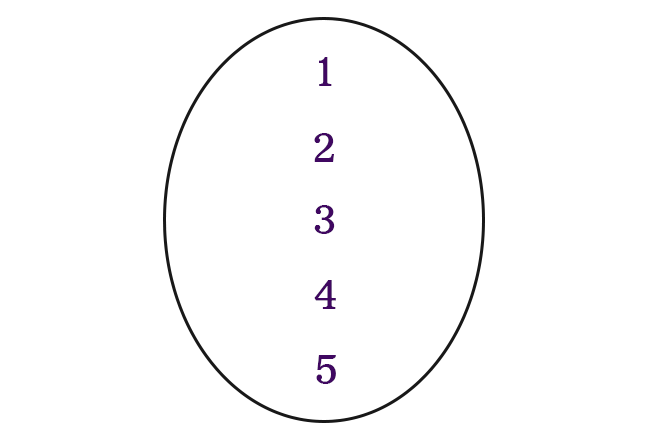# Representation of a Set

A method of expressing a collection of well-defined objects is called the representation of a set.

## Introduction

There is a purpose for introducing the concept of a set in mathematics. Hence, it is essential to express it in a special way in mathematics.

There are two different ways to express a set in mathematics.

1. Graphical representation
2. Mathematical representation

Now, let’s understand the basic representation of a set from understandable examples.

### Graphical representationIn general, the objects are collected in closed packets. The same principle can also be used in set theory to represent the collection of well-defined objects. Therefore, a set can be represented graphically by a closed geometric shape.

There are several closed geometric shapes in geometry. Hence, a set can be represented by any one of the closed geometric shapes.

For example, a circle, a triangle, a square, or any other closed geometric shape.

### Mathematical representation

There are two common problems in expressing the sets in graphical representation.1. Expressing the different sets in either a closed geometric shape or different closed geometric shapes confuse us, and it irritates us.
2. It is not actually convenient for us to express a set more than once in the form of graphical representation in mathematics.

For overcoming the above two issues, the mathematical representation was introduced in set theory. In this method, we represent every set by a name and they are usually denoted by uppercase (or capital) letters.

For example, we have three sets and they are denoted by capital letters $A$, $B$ and $C$. They are called Set $A$, Set $B$ and Set $C$.

A set of first five natural numbers.$1, 2, 3, 4,$ and $5$ are first five natural numbers. Display the first five natural numbers inside any closed geometrical shape for representing this set graphically.

Similarly, write the first five natural numbers with comma separation inside the curly braces.

$\Big\{\, 1, 2, 3, 4, 5 \,\Big\}$

#### Representation

List of the mathematical methods to learn how to express a set in different mathematical approaches.

Latest Math Topics
Jun 26, 2023
Jun 23, 2023

###### Math Questions

The math problems with solutions to learn how to solve a problem.

Learn solutions

Practice now

###### Math Videos

The math videos tutorials with visual graphics to learn every concept.

Watch now

###### Subscribe us

Get the latest math updates from the Math Doubts by subscribing us.